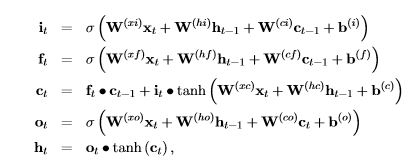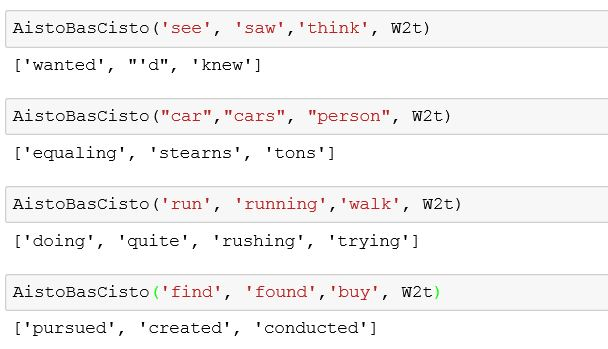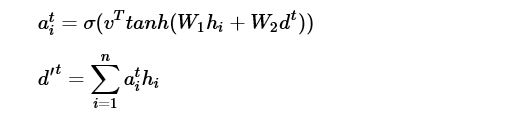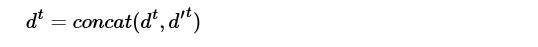# Monthly Archives: March 2016# CNTK’s LSTM and Hallucinating Bloomberg Financial News

One of the many good examples in CNTK is language modeling exercise in Examples/Text/PennTreebank.   The documentation for this one is a bit sparse and the example is really just of a demo for how easy it is to use their “Simple Network Builder” to define a LSTM network and train it with stochastic gradient decent on data from the Penn Treebank Project.   One command starts the learning:

cntk configFile=../Config/rnn.cntk

Doing so trains the network, tests it and saves the model.  However, to see the model data in an easily readable form you need a trivial addition to the configfile: you need to add the following dumpnode command to put a dump file a directory of your choosing.

```dumpnode=[
action = "dumpnode"
modelPath = "\$ModelDir\$/rnn.dnn"
outputFile = "\$OutputDir\$/modeltext/dump"
]
```

This creates a big text file with all the trained data.   To experiment with the trained model, I decided to load it into a python notebook and rebuild the LSTM network from the defining equations.  From the CNTK book those equations areI was pleased to see that the dumped model text had the same W and b tensors names as in the equations, so my job was relatively easy.    I extracted each of the tensors and saved them into a file (I will make these available in Github).   The python code for the LSTM based on the equations above is below.

```def rnn(word, old_h, old_c):
Xvec = getvec(word, E)

i = Sigmoid(np.matmul(WXI, Xvec) +
np.matmul(WHI, old_h) + WCI*old_c + bI)
f = Sigmoid(np.matmul(WXF, Xvec) +
np.matmul(WHF, old_h) + WCF*old_c + bF)

c = f*old_c + i*(np.tanh(np.matmul(WXC, Xvec) +
np.matmul(WHC, old_h) + bC))

o = Sigmoid(np.matmul(WXO, Xvec)+
np.matmul(WHO, old_h)+ (WCO * c)+ bO)

h = o * np.tanh(c)

#extract ordered list of five best possible next words
q = h.copy()
q.shape = (1, 200)
output = np.matmul(q, W2)
outlist = getwordsfromoutput(output)
return h, c, outlist
```

As you can see, this is almost a literal translation of the equations.    The only different is that this has as input a text string for the input word.  However the input to the equations is a vector encoding of the word.  The model generates the encoding matrix E which has the nice property that the ith column of matrix corresponds to the word in the ith position in the vocabulary list.  The function getvec(word, E) takes the embedding tensor E, and looks up the position of the word  in the vocabulary list and returns the column vector of E that corresponds to that word.   The output of one pass through the LSTM cell is the vector h.  This is a compact representation of the words likely to follow the input text to this point.  To convert this back into “vocabulary” space we multiply it by another trained vector W2.  The size of our vocabulary is 10000 and the vector output is that length.  The ith element of output represents the relative likelihood that that ith word is next word to follow the input so far.  Getwordsfromoutput simply returns the top 5 candidate words in order of likelihood.

Before going further, it is worth looking closer at the properties of the word embedding matrices E and W2.   There is a fascinating paper by  Mikolov, Yih and Zweig entitled “Linguistic Regularities in Continuous Space Word Representations” where they suggest that the embedding space for word has several interesting properties.   I decided to investigate that.   Their point is that words that are similar in a linguistic sense will be nearby in the embedding space.   For example, present tense verbs should be near other present tense verbs and singular nouns should be near each other, etc.   I decided to try that.  However, there are two embedding mappings.  One is based on the tensor E and the other based on the W2 tensor.   E has dimension 150 by 10000 and W2 is 200 by 10000.  The difference in dimensionality are because of arbitrary decisions made in defining the hidden layers in the network.  But both represent word imbeddings.  I experimented with both.  I wrote a function getnear(word, M) which takes a word and looks for the 5 most nearby words in the space where M is transpose of either E or W2. (I used cosine distance as the metric.) Verb tense locality and noun plurals worked best in the W2 space as illustrated below.These are only illustrations.  For a deeper statistical analysis look at the Mikolov paper.   A more interesting conjecture from their study was that there may be some linearity in these embedding that might allow one to try simple analogies of the form “A is to B, as C is to __”.   Their idea is that if a, b and c are the vector embeddings of the words A, B and C, then the embedding of “__” may be computed as d = c + (b-a).  So I wrote a little function AistoBasCisto(A, B, C) that does this computation.   In the results I had to delete A, B and C from the candidate answers because they came up often as nearby.   In this case my results were less encouraging.  It worked better with the E space than with W2.   For example, for E we haveAnd for the W2 space the results looked likeAs you can see the “run running walk __” example failed with E but was close, but still incorrect, with W2.

You may wonder why these particular words came up.  The data we used to train the system came from a small subset of the Penn TreeBank collection as provided in the CNTK package.   It is heavily dominated by financial news items.    This explains why the plural of person could be managers or customers.   A larger vocabulary and data collection would be needed to truly test the analogy by linearity conjecture.

#### Now on to hallucinating the financial news.

Now to test the LSTM as a truly recurrent network.   We provide the network with a starting word and let it suggest the next word.  And then we repeat this process constructing a “sentence”.  In the code below we randomly pick one of the top three suggest by the network as the next word.

```c = np.zeros(shape = (200, 1))
h = np.zeros(shape = (200, 1))
output = np.zeros(shape = (10000, 1))
word = 'my'
sentence= word
for _ in range(40):
h, c, outlist = rnn(word, h, c)
word = outlist[randint(0,3)]
sentence = sentence + " " +word
print sentence+"."
```

In this case we start with the word “my” and let it generate a 40 word sentence.  The output is

```my new rules which would create an interest position here unless there should prove   signs of such things too quickly although the market could be done better toward paying further volatility where it would pay cash around again if everybody can.
```

This is a great example of hallucinating financial news. Let’s try it again starting with the word “president”.

```president michael de brown wrote himself against democratic union law which represents an emergency relief agreement during a new york state district or early tuesday before july after a federal government agency created early losses without mr. krenz or perhaps.
```

Now with the word “the”.

```the company reported third-quarter results reflecting a number compared between N barrels including pretax operating loss from a month following fiscal month ending july earlier compared slightly higher while six-month cds increased sharply tuesday after an after-tax loss reflecting a strong.
```

The “sentences” end rather abruptly because of the 40 word limit I set.  If you let it go it will run on until the state vector for the sentence seems to break down.     Try this yourself.  To make it easy to play with this example, I have put the code in GitHub.  The trained model text files are in OneDrive and is a zipped file of about 50MB.

There are many more excellent and fun examples.  Andrej Karpathy has a great blog article showing how RNNs can mimic Shakespeare, or Latex science articles and many more.

## TensorFlow’s seq2seq French Lesson.

One of the most interesting examples in the TensorFlow tutorials is an English to French translator.  As with the CNTK example it was trivial to start the translator learning following the instructions in the tutorial.   After letting this run for about a week, I wanted to see how well it would do.     As with the CNTK example, I created a Jupyter IPython notebook and loaded the trained model.   I will explain how that was done in more detail below but, for now, I will show how we can invoke it to test its translation ability.    This particular trained model was not very big and with a relatively small data set, so I didn’t expect much.    In fact, as you will see, to a French speaker it is a disaster.   On the other hand, it learned more French in a week of training that I did in three semesters of French in college.   (For full disclosure, this was my weakest subject in college and my grade was a hard-fought “C” each semester.)

The code below demonstrates how the model is invoked.   First you have to tokenize the input sentence.  The algorithm uses a system of buckets of fixed sizes to make the training more efficient.  You next find the smallest bucket that can contain your sentence and convert this to the input vector list needed by the model.   The step function takes a Tensorflow session, the input vector list and a null list of decoder inputs (to be explained later) and generates a list of vectors as outputs.  Each vector represents the likelihood that individual vocabulary words are the correct word at that point in the translated sentence.   We pick the most likely and print the sentence.

```sentence = " I am not the president of France. "

token_ids = data_utils.sentence_to_token_ids(sentence, en_vocab)
# Which bucket does it belong to?
bucket_id = min([b for b in xrange(len(_buckets))
if _buckets[b] > len(token_ids)])
# Get a 1-element batch to feed the sentence to the model.
encoder_inputs, decoder_inputs, target_weights =
model.get_batch({bucket_id: [(token_ids, [])]}, bucket_id)

_, _, output_logits = model.step(sess, encoder_inputs, decoder_inputs,
target_weights, bucket_id, True)

outputs = [int(np.argmax(logit, axis=1)) for logit in output_logits]
print(" ".join([rev_fr_vocab[output] for output in outputs]))

Je ne suis pas le président de la France .
```

“In which city does the president of France live?”

I get

“Dans quelle ville le président de la France ?”.

This is not exactly correct.    If I feed this into Google translate and ask what this means in English I get “In which city the President of France?”.   If I give it this one,

What is the name of a good restaurant?

The system responds with

Quel est le nom d’une bonne bonne bonne ?”

Which translates back to “What is the name of a good good good?”.  Probably not very helpful on the streets of Paris.   It turns out restaurant is not in the tiny training vocabulary used here.   Finally, given this sentence

” The article stated that the President of the United States is here today. “

The translator returned

Le paragraphe a indiqué que le président des États-Unis est aujourd ‘ hui aujourd ‘ hui .”

The end of this reply is “is today today”.    As I said, this is still much better than I could do with my college French.   However, as you can see from the previous two examples, our little translator runs out of gas at the end of sentences and tends to repeat itself.   You should try this yourself.   I have put the notebook file in github or you can execute these directly from the Tensorflow python code.   All you need to do is train the model from TensorFlow and run the notebook with the path to the model output directory.

While loading and using the trained model was easy and fun, understanding the seq2seq model used in this example takes a bit of work.   So this part of this article will get a bit more technical.

The TensorFlow translate program is based on a sequence-to-sequence model constructed from more primitive recurrent neural nets.   By sequence-to-sequence we mean a network that takes a sequence as input and produces a sequence as output.   It consists of two parts: an “encoder RNN” and a “decoder RNN” as shown in Figure 1 below.Figure 1.   A sequence-to-sequence RNN English to French translator with the encoder and decoder unrolled to show the flow of messages.

In this figure the RNNs are “unrolled” to show the flow of messages.  The state vector at the end of the encoder is a vector embedding of the input sentence.   This state vector is used to start the decoder along with a “GO” token.  The diagram shows the network after it has been trained.   During training the inputs to the decoder are the French version of the English sentences.   I won’t talk about the training here because is enough to try to understand how this works.  Before I go any further I want to point you to some important papers.  Sutskever, Vinyals and Le published an early important paper on sequence to sequence models that is worth reading.

To understand how it is built the network we need to dig into the code a bit. The building blocks are a set of classes of base type RNNCell with specializations

1. BasicRNNCell
2. GRUCell
3. BasicLSTMCell
4. LSTMCell
5. OutputProjectionWrapper
6. InputProjectionWrapper
7. EmbeddingWrapper
8. MultiRNNCell

The ones we will see used here are GRUCell, MultiRNNCell and EmbeddingWrapper.   We discussed LSTMCell in our previous article but we need to look at GRUCell here because that is the one used in the example.   The GRUCell is a “Gated Recurrent Unit” invented by Cho et. al.  in “Learning Phrase Representations using RNN Encoder–Decoder for Statistical Machine Translation”.  The “gated” phrase comes from the way the output is defined as coming mostly from the previous state or from a combination with the new input.   The diagram below tries to explain this a bit better.Figure 2.   GRU wiring diagram

It also helps to see it in terms of the defining equations.The quantity ut  is a gate vector.  Recall the sigmoid function switches sharply between one and zero.  So when ut is one then h is just a copy of the old h and we are ignoring the input x it is based on the value ct.   The gate rt is determines how much of the old state goes into defining the value of ct.   To understand how this is encoded in TensorFlow you need to understand the function.

```linear(args, output_size, bias, bias_start=0.0, scope=None)
```

where args is a list of tensors each of size batch x n .   Linear computes sum_i(args[i] * W[i]) + bias where W is a list of matrix variables of size n x outputsize and bias is a variable of size outputsize.   In the equations above we have represented linear algebra as a matrix times a column vector.   Tensorflow uses the transpose notation:   row vector on the left times the transpose of the matrix.   So in linear the args are a list of row vectors.   Where is the matrix W and offset b?  This is fetched from memory based on the variable current scope, because W and b are variable tensors that are learned values.   If you look at the first two equations above, you will see they are almost identical.   In fact, we can write them asIf you transpose the last one from column form into row form you can now compute both with one invocation of the linear function.   The code for the GRUCell is below.   As you can see they have encoded one pass through the GRU cell with only two matrix vector multiplies.   You can also see that the way the variable scope is used to pick out the W’s for the gates and the W for the state/output.  Another point to remember that an invocation of the “__call__ function operator does not cause the tensor to execute the operation, rather it builds the graph.

```class GRUCell(RNNCell):
def __init__(self, num_units):
self._num_units = num_units
... stuff deleted ....
def __call__(self, inputs, state, scope=None):
with vs.variable_scope(scope or type(self).__name__):
with vs.variable_scope("Gates"):  # Reset gate and update gate.
# We start with bias of 1.0 to not reset and not udpate.
r, u = array_ops.split(1, 2, linear([inputs, state],
2 * self._num_units, True, 1.0))
r, u = sigmoid(r), sigmoid(u)
with vs.variable_scope("Candidate"):
c = tanh(linear([inputs, r * state], self._num_units, True))
new_h = u * state + (1 - u) * c
return new_h, new_h
```

The top level class we invoke for building our model is seq2seqModel.    When we create an instance of this class it sets in motion a set of flowgraph building steps.  I am going to skip over a lot of stuff and try to give you the big picture.  The first graph building step in the initialization of an instance of this object is

```# Create the internal multi-layer cell for our RNN.
single_cell = rnn_cell.GRUCell(size)
…
if num_layers > 1:
cell = rnn_cell.MultiRNNCell([single_cell] * num_layers)
```

As you can see we are creating a GRU cell graph generator instance and making a list of num_layers of this object and passing that to the constructor for MultiRNNCell.   In our case, num_layers has been set to 2.   MultiRNNCell is pretty easy to understand.   It builds a graph consisting of a stack of (in this case) GRU cells where the output state vector of each level is fed to the input of the level above it.  This new compound cell has an output that is the state of the top sub-cell and whose output state is the concatenation of the output states of all the sub-cells.

The next part is not so easy to follow.    We will take our MultiRNNCell graph builder and use it to create and encoder and a special decoder.    But first we must make a short digression.

## Paying Attention

There is a problem that is encountered in the sequence-to-sequence model.   The encoder encodes the entire sentence into a state vector which is used by the decoder as its input.    That state vector is an abstract representation of our entire sentence as a single point in a very high dimensional space.    The decoder has been trained to use that point as a starting point to unroll a translated version of the sentence.   I find the fact that it works at all to be rather remarkable.   It is as if the decoder takes the English state vector and transforms it into a similar point in “French” space.

Unfortunately, the longer the input sentence, the more difficult it is to decode it.   How much information can we pack into one point?   The problem is that at each decoding step we need a little bit more information than is provided by the state vector as it passes through the decoder loop.   The idea used here is to help the decoder by providing it a bit of focus derived from the input sequence at each stage of the decoder loop.  This is generally referred to as “attention”, as in “at this step of decoding please pay attention to what the encoder was doing here”.   Bahdanau, Cho and Bengio had an early paper about this that used a bidirectional pass over the input sequence. As they put it, they wanted to “automatically (soft-)search for parts of a source sentence that are relevant to predicting a target word”.  (Denny Britz has a lovely blog article about attention and describes several fascinating applications.  It is  well worth  reading.) The mechanism for attention used in the TensorFlow example is based on a paper by Vinyals et. al. and we will follow that one here.   The key idea is rather than take the single final state vector from the encoder, let’s collect the state vectors at each stage of the encoder.   Following Vinyals, let the encoder state vectors for each input word beAnd let the decoder state vectors beThen for each decoder time step t computeWhere the Ws are learned matrices and v is a learned vector.    Then as the input to the t+1 state vector of the decoder we use the concatenationThe idea is this new state vector at time t+1 puts much more focus on the corresponding words in in the encoder string. This all happens in a function called seq2seq.attention_decoder that is called in another constructor function seq2seq.embedding_attention_seq2seq that wraps and an embedding around a graph generated by our MultiCellRNN graph builder to generate the final decoder graph.   These graphs are all stitched together in the Seq2SeqModel constructor.  It is fair to say that there are many levels of abstraction here that are used to build the decoder and link it to the encoder.  I am leaving out many details that are critical for the training such as the part that implements the bucket handler.   The final graph, in its most abstract form is pictured below in figure 3.Figure 3.  The Translate.py sequence to sequence translator is based on a two level GRU cell encoder and an attention-augmented two level GRU cell decoder.  The input English is entered in reverse order as an optimization

## Final Thoughts

As I have said above, I have not included all the details of how the seq2seq translator is put together, but I tried to include the highlights that I found most interesting.   I encourage you to dive into the code and discover the rest.   You will likely find some errors in what I described above.   If so, please let me know.

There is really a lot of exciting results that have come out in the last few years relating to RNNs.   For example, Lei Ba, Mnih and Kavukcuoglu demonstrated that RNNs with attention can be applied to interesting image analysis challenges, such as reading the house number from a street scene.   In “Teaching Machines to Read and Comprehend” Hermann et. al. excellent paper demonstrate the use of an attentive RNN build to answer simple questions about text.   I personally don’t think any RNN can pass a Turing test yet, so it ain’t A.I.  But these little statistical machines are certainly wonderful mimics and they can speak better French than I.